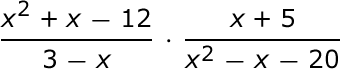# Rational Expressions Chapter Exam

Free Practice Test Instructions:

Choose your answer to the question and click 'Continue' to see how you did. Then click 'Next Question' to answer the next question. When you have completed the free practice test, click 'View Results' to see your results. Good luck!

#### Question 2 2. The expression below can be simplified to which of the following expressions?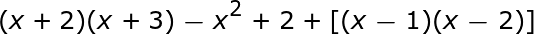#### Question 4 4. Which of the following is equivalent to this expression: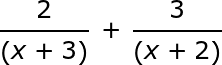#### Question 5 5. For following equation, x is equal to which of the following?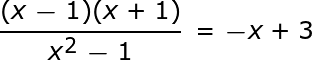#### Question 6 6. The expression below can be simplified to which of the following expressions?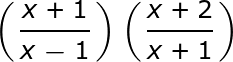#### Question 7 7. The expression below can be simplified to which of the following expressions?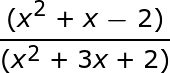#### Question 8 8. Solve for x in the following equation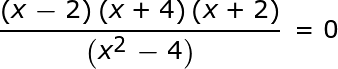#### Question 10 10. The equation below can be simplified to which of the following?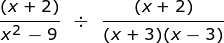#### Question 11 11. The expression below can be simplified to which of the following expressions?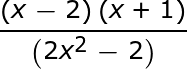#### Question 12 12. What is the least common denominator for the following expression?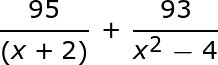#### Question 15 15. Multiply the following rational expressions.